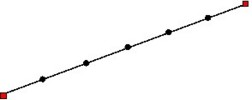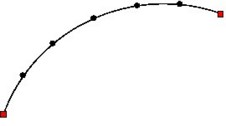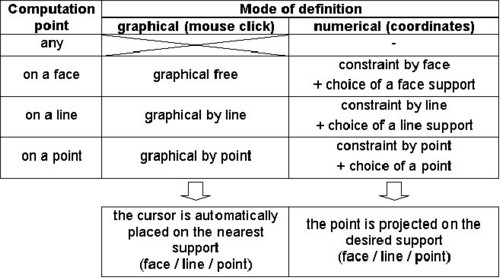## Definition

A path is a line support, on which the user can evaluate a spatial quantity.

## Use

A path is used to plot 2D spatial curves.

## Geometric shapes

The path can be as follows:

• rectilinear (straight segment)
• curvilinear (arc of circle, helicoïdal line)

## Mesh

The mesh of a path is based on a user-defined discretization: computation points are regularly spaced on the line of the support.## Types of path

Types of path are as follows:

Type of path Shape of path Definition
path defined by two points

straight segment /

arc of circle

helicoïdal line

two points *

(graphical or numerical capture)

arc defined by three points arc of circle

three points *

(graphical or numerical capture)

arc defined by center, radius and angles arc of circle

center coordinates,

starting angle and ending angle

parametrized path

straight segment /

arc of circle

helicoïdal line

parameter functions
Note: * To capture points, the user may lean on one of the methods presented in the table below.## Parameter setting

The user can use geometric parameters to define the path by the numerical mode.